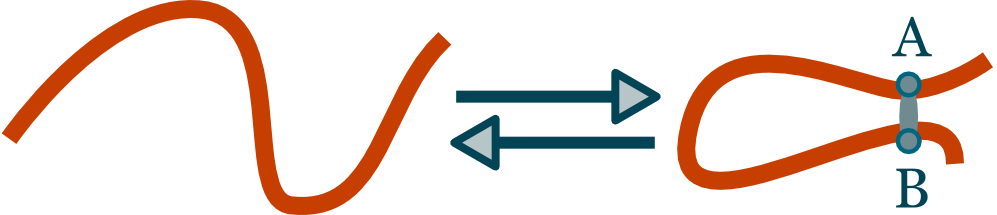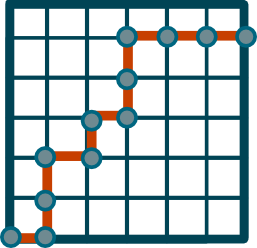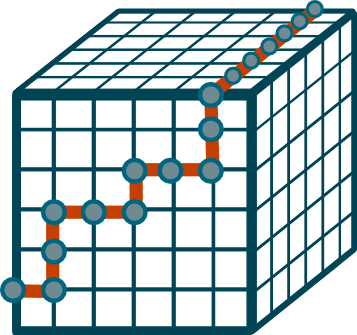# What if DNA Lived on a Lattice?

These are my notes based on the lecture by Dr. Zoie Raputi on June 10th, 2008. Please email me with corrections if mistakes are found.

In this talk the presenter simplifies the DNA model by assuming that they live on a lattice. After defining legal local moves, the presenter is interested in the speedup achieved if such moves are allowed to be executed in parallel.

# The DNA

DNA is the blueprint that instructs the cells how to replicate. It is composed of a combination of phosphates and sugar, with bases that, for simplicity, reside on top of the sugars. Such bases are called monomers and come in four types — Adenine, Thymine, Guanine, and Cytosine. The two basis Adenine and Thymine bond to create a weak pair composed of two hydrogen bonds. The stronger pair is composed of 3 hydrogen bonds and is composed of Guanine and Cytosine.

It is known from experiments that if the DNA is single stranded and a periodic, then it can loop onto itself. If such loops occur, then they are called either hairpins or stem-loop structures. It is worth mentioning that loops are not a strange phenomenon in DNA, as they only lasts for a few milliseconds — although some believe that continuous oscillation occurs. The figure bellow shows a single stranded DNA in open (or coil) state on the left, and a strand of DNA in closed (or native) state on the right. These correspond to the kinetics of the DNA strand.# The Lattice

The presenter simplifies the DNA onto a lattice considering both a cubical and hexagonal lattice in 2 and 3 space. She then defines what local moves and configurations are legal, and which are not. Such moves are influenced by how DNA behaves at the microscopic level. One constraint is the so called stiffness constrain. It states that the angle between two consecutive bond (edges in the lattice) must be larger than $120^\circ$.

## Square Lattice

The square lattice is the simplest lattice to discritize the DNA on. There are only two directions $x_1$ and $x_2$ (the inverse directions $x^{-1} = x_1$ and $y^{-1} = y_1$ being trivial due to the symmetry of the square). Since no restriction is placed on the configuration, all words are considered legal.## Cubic Lattice

The cubical lattice contains three directions $x, y,$ and $z$. The inverses are again trivial to deduce, and, since no restriction is placed on the placement of the DNA, all words are legal.If we consider the 2-dimensional cubic lattice where the directions $x, y,$ and $z$ form $0^\circ, 60^\circ$, or $120^\circ$. In this case some words are not allowed since they conflict with our stiffness constraint. One can enumerate all the legal words in this lattice and get:

xy      yx
yz      zy


It is easy to see how one can get this if we represent $x$, $y$ and $z$ as vectors in $\mathbb{R}^2$. $x = \langle 1, 0 \rangle$, $y = \langle \frac{1}{2}, \frac{\sqrt{3}}{2} \rangle$, and $z = \langle \frac{-1}{2}, \frac{\sqrt{3}}{2} \rangle$

If we consider the 3-dimensional cubical lattice, there are are a total of 6 directions $x_i$ for $i = 0, 1, \cdots, 6$. The first three are planer and are the same as the 2-dimensional cubical lattice case. The other three spring out of two dimensions and are $\langle \frac{-1}{2}, \frac{-1}{2\sqrt{3}}, \frac{\sqrt{2}}{\sqrt{3}} \rangle$, $\langle \frac{1}{2}, \frac{-1}{2\sqrt{3}}, \frac{\sqrt{2}}{\sqrt{3}} \rangle$, and $\langle 0, \frac{1}{\sqrt{3}}, \frac{\sqrt{2}}{\sqrt{3}} \rangle$ .